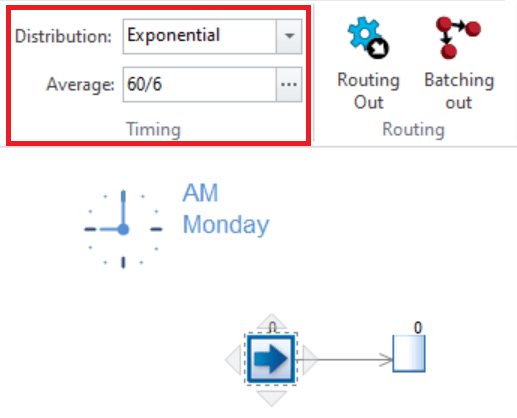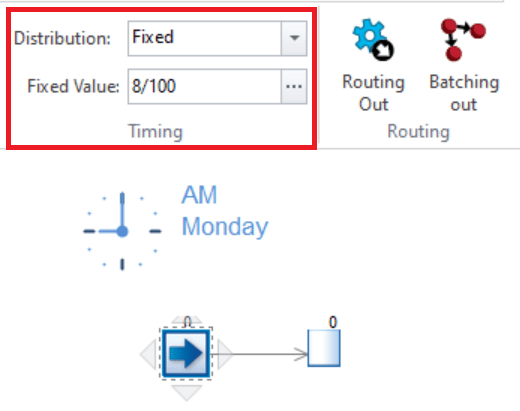# Simul8 Tip – What’s The Difference Between Arrival Rates and Inter Arrival Times?

### We have a really great tip for you today. We’ve heard that sometimes users get confused between arrival rates and inter-arrival times.

Well, let’s clarify this for you; the inter arrival time is a key option in the Start Point of any simulation, therefore it’s really important that users know the difference before they start simulating.

### Whats the difference between arrival rates and inter arrival times?

• The arrival rate is the number of arrivals per unit of time.
• The inter arrival time is the time between each arrival into the system and the next.

When building a simulation, one of the main inputs concerns the arrival of work items at a Start Point. That is, the number of arrivals, e.g., how many per hour or day etc., and the randomness with which they arrive. For instance, do arrivals occur fairly predictably, such as (evenly distributed or even fixed) every 10 minutes, or less so, say according to an exponential distribution?

All this can be set, when a Start Point is selected, in the dialog shown below.Now, from experience we know that arrival data comes in different forms. The default option in the Start Point dialog is to use the inter-arrival time, i.e., an arrival occurs every n minutes. For example, in the above picture on average (following an exponential arrival pattern) we see a work item coming into the simulation every 10 minutes. You can change the distribution, for instance to a fixed one, if an arrival occurs exactly every 10 minutes. Therefore, the inter arrival time is the time between each arrival into the system and the next. If this is your preferred way of specifying a Start Point, great, go ahead.

Alternatively, our users often also consider the arrival rate. Sometimes, this is due to the way their data is being collected or simply a more intuitive understanding about arrivals. The arrival rate is simply how many arrivals occur in a specified time interval (e.g., per hour, day etc.). It can be derived through the equation of arrival rate = 1/inter-arrival time. At a Start Point, this is useful to know as this equation can be inverted and used directly as an input. Therefore, you can directly set a Start Point input to be [1/number of arrivals]*length of time interval.

Let’s clarify this! So, if you know that you have 6 arrivals per hour and your simulation runs in minutes, then Simul8 allows for directly setting the Start Point input to be a formula, such as [1/6]*60 or likewise 60/6. Of course, in this simple example this corresponds to an arrival every 10 minutes, but it is important to note that you can input arrival rates that way directly as shown below.This can be useful if the number of arrivals changes dynamically, for example daily, and you refer to a variable instead of a fixed number of 6 in the above. Or simply, if you want to save yourself from the hassle of calculating the arrival rate manually.

If you run your simulation in other time units, say in hours, or are interested in the number of arrivals over a different time interval, e.g., per day (instead of hour), the above can easily adopted. For instance, if you have an arrival rate of 100 arrivals per an 8-hour day and run your simulation in hours, you can input the following.### So, how do you calculate arrival rates and inter arrival times?

The arrival rate is calculated from the following equation;

arrival rate = 1/inter arrival time

The neat trick with this equation is that if you invert the arrival rate your answer is the inter arrival time which can then be used in your simulation.

inter arrival time = 1/arrival rate

For example;

If 12 customers enter a store per hour, the time between each arrival is;

inter arrival time = 1/arrival rate

= 1/12

= 0.083(hours)

= 0.083 x 60 minutes

= 5 (minutes)

Therefore from the arrival rate of 12 per hour, the time between each arrival is 5 minutes.

We hope you found this Simul8 tip useful!